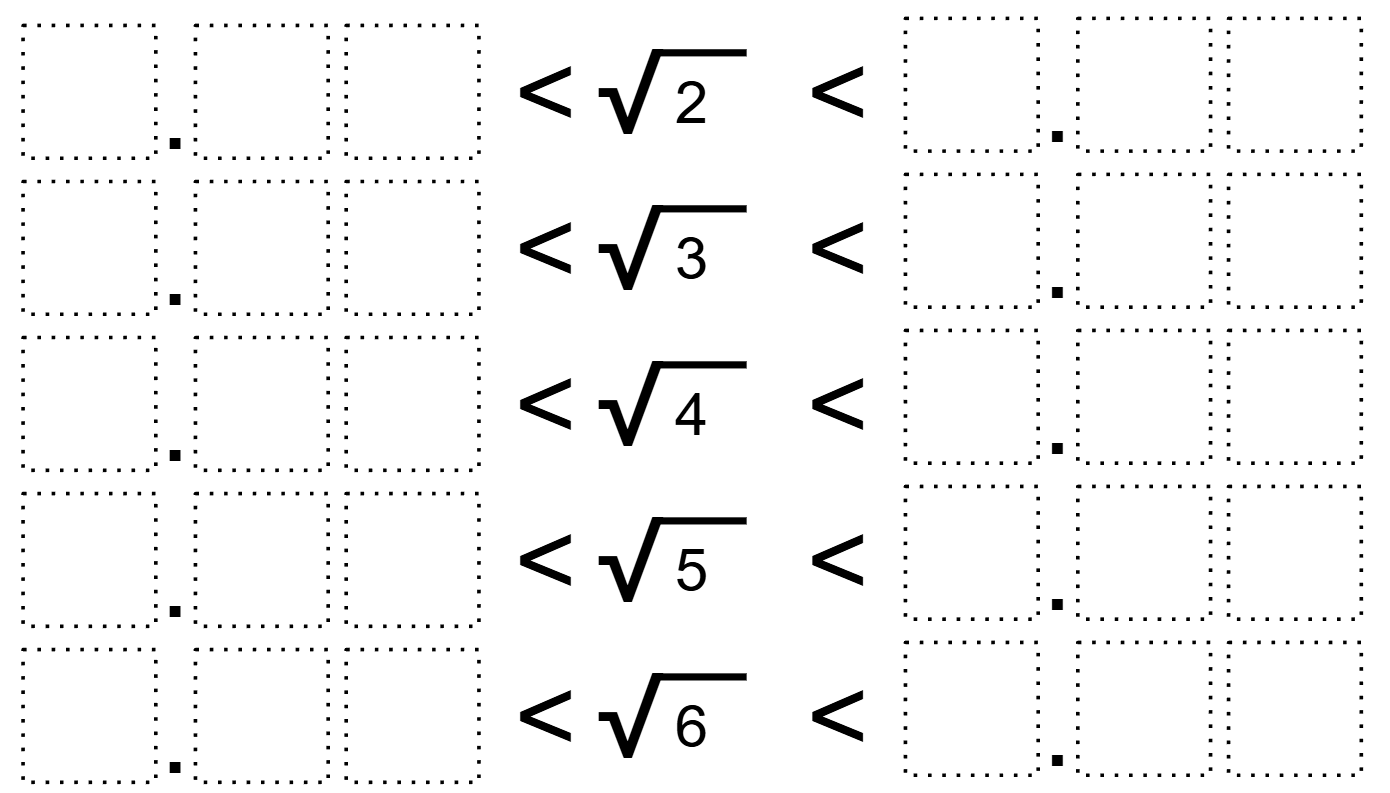# Decimal Approximations of Roots

Directions: Using the digits 1 to 6, at most one time each, fill in the boxes to create a true statement with the smallest possible interval:### Hint

What place value will impact the interval the most?

1.36 < √2 < 2.45
1.65 < √3 < 2.34
1.65 < √4 < 2.34
2.16 < √5 < 3.45
2.41 < √6 < 3.56

Source: Bryan Anderson

## Exponential Powers

Directions: Using the digits 0 to 9 at most one time each, place a digit …

1.2._
1.36 < V2 < 2.04
_
1.73 < V3 < 2.04
_
1.65 < V4 < 2.03
_
2.16 < V5 < 3.04
_
2.41 < V6 < 3.05

PS: The google form has a mistake. It says what number did you choose, then gives us the options A, B, C, D.

3.1 V2 2.50
4.5.